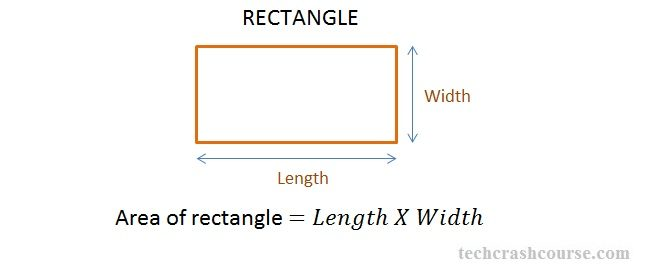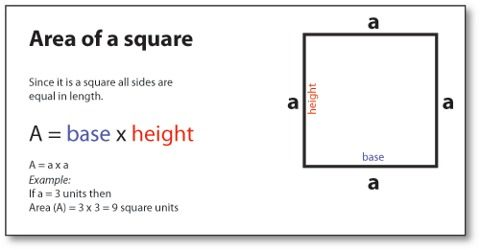• No products in the cart.

# SCI/P/5: MEASUREMENT

##### this is unit in the science subject to enable pupils understand different aspects in science

It is the process of finding out how long, short, big, small, heavy or light an object is.

Mass

It is the amount or quantity of matter in an object.

It is measured in grams (g), kg (kilograms).

NB: Its standard unit is kg.

Gravity: Is the force of the earth that pulls down objects.or Is the force of attraction that objects have on one another because of their masses.

NB On earth the gravitational force acting on mass is 10N

The size of the force becomes smaller as the object moves further from the surface of the earth.

Length

• It is the distance between two point
• It is measured in metres (m), centimeters (cm) Hecto metres (hm), millimetrees (mm, decametre (Dm) decimeters (dm).

NB: The standard units for length are Metres

Instruments used to measure length

1. Tape measures
2. Metre rulers
3. Foot rulers
4. Sticks
5. Strings
6. Strides

A line segment: Is a line between two points.

Activity

Learners draw line segments of different length.

a). 4cm                b) 6cm        c)   8cm                 d) 14cm

AREA

It is the total space covered by an object.

It is measured in Square Units cm2, m2, dm2, km2.

Area of a rectangleThe width is the shorter side of a rectangle

The length is the longer side of a rectangle

Finding area

Area is calculated by multiplying the longest side (length) by the shorter side (width). Length is represented by letter L and with is represented by letter W.

Find the area of the figure below

Area = Length x width=              sq unitsNB: A regular rectangle has two opposite sides equal. Basic units for area are square units e.g. cm2, m2, km2 etc

Activity

Area of a square

A square has all its sides equal

Area = s x s

=  S2Area = s x s

= 4cm x 4cm

= 16cm2

Volume

It is the space occupied by an object

It is measured in cubic units (cc, cm2, mm3, m3))

Types of shaped objects

There are two types of shaped objects namely;

1. Regular shaped objects
2. Irregular shaped objects

Regular objects

They are objects with specific (definite) shapes e.g. cuboids, bricks, blocks, tins, rectangles, square etcFinding volume of regular objects

Find the volume of the figure above.

Irregular shaped objects

These are objects which don’t have proper or well defined shape e.g. stones, keys, needles, shoes, sweet potato tuber, cassava tuber etc

Finding volume of regular shaped objects

Displacement method

Is the method used to find the volume of irregular objects.

Instruments used to measure the volume of irregular objects

1. Measuring cylinder
2. An over flow can or Eureka can

It is called the displacement method because irregular object displaces the amount of water equal to its volume.

1. Using a measuring cylinder

Requirements;

Water, measuring cylinder, stone or sweet potato etc

Procedure

Step I

Pour water into a measuring cylinder about half full and record the volume of the water.

Step II

Gently lower the irregular object tied on a string into the measuring cylinder.

Step III

Record the final level of the water in the measuring cylinder.

Step IV

Subtract the first level from the final level.

V = Final level – 1st level

V = 10cc – 5cc

V = 5cc

NB

The volume of the irregular object is equal to the volume of the displaced water.

Using an over flow can (Eureka can)

Step I

Pour water in the can up to the level of the spout

Step II

Put the irregular object tied on a thread gently in the can.

Step III

Collect the water that pours out of the can in a measuring cylinder

1. What is the volume of the stone?

10cc

1. What is the use of the string (thread) in the experiment above? To gently lower the stone into the water and avoid it from splashing.

Weight and mass

Weight is the pull of an object towards the earth by the force of gravity. The weight of an object is determined by the following;

Size of the object

Material from which an object is made

The pull of the force of gravity

The basic unit for weight is Newtons (N).

Mass is the quantity of matter contained in an object. Mass is constant i.e. it doesn’t change and it doesn’t depend on the pull of gravity.

The units for mass are grammes (g) or kilogrammes (kg). weight and mass are both measured using the following instruments.

• Beam balance
• Spring balance
• Set of scales
• Scale balance

Density

Density is mass per unit volume. The density of an object is found after knowing its mass and its volume. The fomular for finding density is;

Density (D) = mass (m) or weight                          D =    M

Volume                                                  V

The units for density are grammes per cubic units i.e.

Grammes per cubic centimetres (g/cc)/ g/cm3

Grammes per cubic mililitres g/ml3

Densities of liquids

Liquids have different densities.

Density of liquids is measured by an instrument called a hydrometer.

Finding density

Example: Find the density of an object of mass 150g and volume of 30cc.

Difference between weight and mass

1. Mass does not change from place to place while weight changes.
2. Mass is the amount of matter in an object while weight is the force of gravity exerted on an object.
3. Mass is measured in kilograms while weight is measured in Newton (N).

Floating objects

Floating is when an object stays on top when thrown on water.

Objects float on water because they are less dense than the density of water or objects float because there density less dense than water.

When an object floats in a fluid, two forces act on it; its own weight and upthrust force of the fluid.Examples of floating objects

Cork

Plastic

Boats

Sponge

Feather

Leaves

Soft dry wood

Paper

Petrol, paraffin

Sinking

It is when an object thrown on water goes to the bottom of the water.

Objects sink because they are more dense than water.

Examples of sinking objects

Stones

Sand

Soil

Metal

Glass

Nails

Coins

Pins

Clay

Keys

NB: Any sinking objects displaces water equal to its volume while a floating object displaces water equal to its weight.